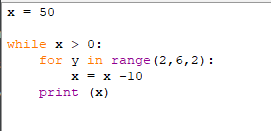# Exercises to Practice (Module 9)

while Loops

Exercise 1

Take a look at the following section of a Python program:Α. How many times will the print command be executed?

B. How many times will the command x = x-10 will be executed;

Exercise 2

Convert the following program portion into an equivalent using the for instruction.Exercise 3

Write a program that will display the numbers 1, 2, 3, 4 to 1000 using the repetition structure while

Exercise 4

Write a program that will read from the keyboard 10 integers, calculate their product, and display it.

Exercise 5

Write a program that reads 10 numbers from keyboard and displays the count of positive, negative, and zero elements entered.

Exercise 6

Write a program that accepts a positive integer and inverts its digits. (Example: 456 becomes 654)

Άσκηση 7

Write a program that accepts an integer as an input and returns the sum of its digits (ex. Input 56, returns 5 + 6 = 11)

Exercise 8

Write a program that accepts an unknown number of integers until their total exceeds 500.

In the end, display the count of numbers that has been accepted.

Exercise 9

Write a program that will read random numbers from the keyboard until it gets the number 0. The program will calculate and print:

Α) How many numbers were accepted (do not count 0),

Β) The count of even numbers

Γ) Sum and average of odd numbers.

Exercise 10

Write a program that calculates and prints the average of the odd four-digit positive integers.

ChallengeOn a ferry, there are First Class tickets (code A) for 50 \$ each and B Class tickets (code B) for \$ 20 each. The maximum number allowed passengers are 400. Please assume that the tickets are sold out for this trip. Write a program in Python, which:
1) Accepts the ticket category (code A or B) for each passenger.
2) Display the number of passengers in the first class.
3) Display the ship's total revenue.